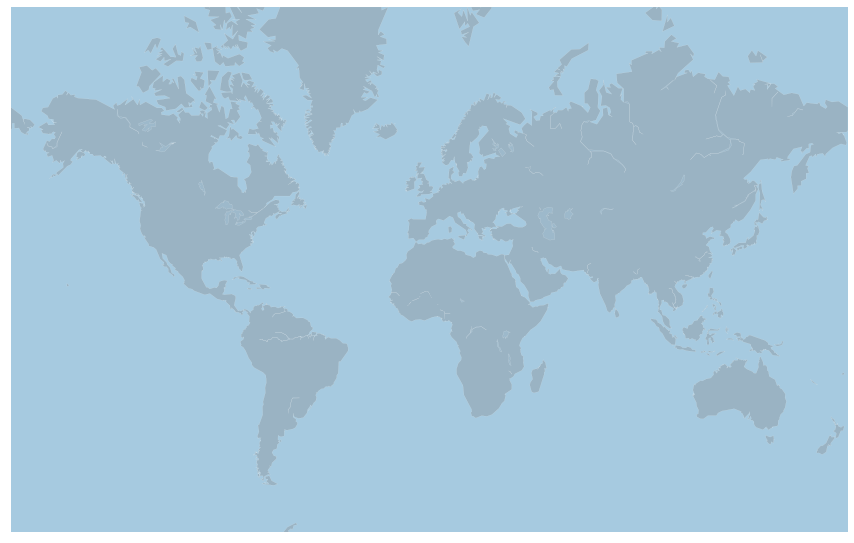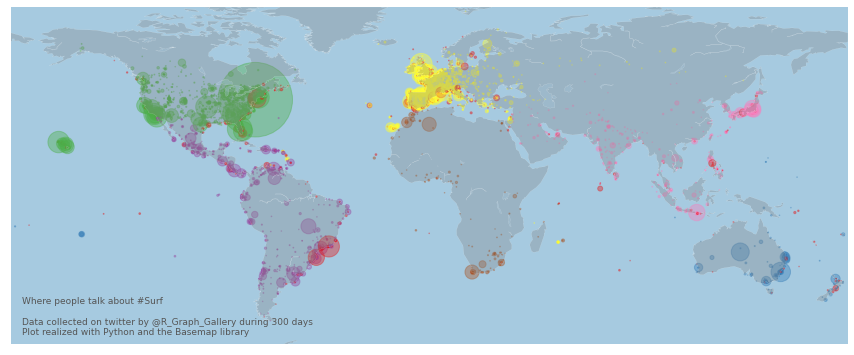I've built a bot that harvested every tweet containing the hashtags `#surf`, `#kitesurf` and `#windsurf` for a couple of days. Those tweets all have a location with geographic coordinates. You can read more about this side project here.

The dataset is stored on github. Let's load it using `pandas`. Note that I've already aggregated the dataset per location. So for each location, I have a `n` column that tells the number of tweets.

``````# Libraries
import pandas as pd

# read the data (on the web)

# Check the first 2 rows

``````
homelon homelat homecontinent n
0 -178.12 -14.29 Australia 10
1 -172.10 -13.76 NaN 2

## 📍 Background map

As explained in the background map section of the gallery, there are several way to build a background map with Python. Here I suggest to use the `basemap` library that provides boundaries for every country:

``````# Basemap library
from mpl_toolkits.basemap import Basemap
import matplotlib.pyplot as plt

# Set the dimension of the figure
plt.rcParams["figure.figsize"]=15,10;

# Make the background map
m=Basemap(llcrnrlon=-180, llcrnrlat=-65, urcrnrlon=180, urcrnrlat=80, projection='merc');
m.drawmapboundary(fill_color='#A6CAE0', linewidth=0);
m.fillcontinents(color='grey', alpha=0.3);
m.drawcoastlines(linewidth=0.1, color="white");

``````## ⭕ Bubble map

Let's add each data point on the map thanks to the `scatter()` function. The scatter() funcion is described extensively in the scatterplot section of the gallery. `x` and `y` coordinates are longitude and latitude respectively. `s` is the size of each circle, it is mapped to the `n` color of the data frame.

``````# Make the background map
m=Basemap(llcrnrlon=-180, llcrnrlat=-65, urcrnrlon=180, urcrnrlat=80)
m.drawmapboundary(fill_color='#A6CAE0', linewidth=0)
m.fillcontinents(color='grey', alpha=0.3)
m.drawcoastlines(linewidth=0.1, color="white")

# prepare a color for each point depending on the continent.
data['labels_enc'] = pd.factorize(data['homecontinent'])

# Add a point per position
m.scatter(
x=data['homelon'],
y=data['homelat'],
s=data['n']/6,
alpha=0.4,
c=data['labels_enc'],
cmap="Set1"
)

# copyright and source data info
plt.text( -175, -62,'Where people talk about #Surf\n\nData collected on twitter by @R_Graph_Gallery during 300 days\nPlot realized with Python and the Basemap library', ha='left', va='bottom', size=9, color='#555555' );

``````Note: I can't use the `mercator` projection here. When I do, the circles coordinates are not recognized properly anymore. Please let me know if you have a fix!

## Contact & Edit

👋 This document is a work by Yan Holtz. You can contribute on github, send me a feedback on twitter or subscribe to the newsletter to know when new examples are published! 🔥